xMolecular term symbolEncyclopedia
In molecular physics
Molecular physics
Molecular physics is the study of the physical properties of molecules, the chemical bonds between atoms as well as the molecular dynamics. Its most important experimental techniques are the various types of spectroscopy...

, the molecular term symbol is a shorthand expression of the group representation
Group representation
In the mathematical field of representation theory, group representations describe abstract groups in terms of linear transformations of vector spaces; in particular, they can be used to represent group elements as matrices so that the group operation can be represented by matrix multiplication...

and angular momenta
Angular momentum
In physics, angular momentum, moment of momentum, or rotational momentum is a conserved vector quantity that can be used to describe the overall state of a physical system...

that characterize the state of a molecule
Molecule
A molecule is an electrically neutral group of at least two atoms held together by covalent chemical bonds. Molecules are distinguished from ions by their electrical charge...

, i.e. its electronic quantum state which is an eigenstate of the electronic molecular Hamiltonian. It is the equivalent of the term symbol
Term symbol
In quantum mechanics, the Russell-Saunders term symbol is an abbreviated description of the angular momentum quantum numbers in a multi-electron atom. It is related with the energy level of a given electron configuration. LS coupling is assumed...

for the atomic case. However, the following presentation is restricted to the case of homonuclear diatomic
Diatomic
Diatomic molecules are molecules composed only of two atoms, of either the same or different chemical elements. The prefix di- means two in Greek. Common diatomic molecules are hydrogen , nitrogen , oxygen , and carbon monoxide . Seven elements exist in the diatomic state in the liquid and solid...

molecules, or symmetric
Symmetry group
The symmetry group of an object is the group of all isometries under which it is invariant with composition as the operation...

molecules with an inversion centre. For heteronuclear diatomic molecules, the u/g symbol does not correspond to any exact symmetry of the electronic molecular Hamiltonian. In the case of less symmetric molecules the molecular term symbol contains the symbol of the group representation
Group representation
In the mathematical field of representation theory, group representations describe abstract groups in terms of linear transformations of vector spaces; in particular, they can be used to represent group elements as matrices so that the group operation can be represented by matrix multiplication...

to which the molecular electronic state belongs.

It has the general form: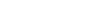where
• S is the total spin quantum number
Spin quantum number
In atomic physics, the spin quantum number is a quantum number that parameterizes the intrinsic angular momentum of a given particle...

• Λ is the projection of the orbital angular momentum along the internuclear axis
• Ω is the projection of the total angular momentum along the internuclear axis
• u/g is the parity
• +/− is the reflection symmetry along an arbitrary plane containing the internuclear axis

## Λ quantum number

For atoms, we use S, L, J and MJ to characterize a given state. In linear molecules, however, the lack of spherical symmetry destroys the relationship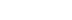, so L ceases to be a good quantum number
Good quantum number
In quantum mechanics, given a particular Hamiltonian H and an operator O with corresponding eigenvalues and eigenvectors given by O|q_j\rangle=q_j|q_j\rangle then the physical quantities q_j are said to be "good quantum numbers" if every eigenvector |q_j\rangle remains an eigenvector of O with the...

. A new set of operators
Operator (physics)
In physics, an operator is a function acting on the space of physical states. As a resultof its application on a physical state, another physical state is obtained, very often along withsome extra relevant information....

have to be used instead: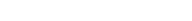, where the z-axis is defined along the internuclear axis of the molecule. Since these operators commute with each other and with the Hamiltonian
Hamiltonian (quantum mechanics)
In quantum mechanics, the Hamiltonian H, also Ȟ or Ĥ, is the operator corresponding to the total energy of the system. Its spectrum is the set of possible outcomes when one measures the total energy of a system...

on the limit of negligible spin-orbit coupling, their eigenvalues may be used to describe a molecule state through the quantum numbers S, MS, ML and MJ.

The cylindrical symmetry of a linear molecule ensures that positive and negative values of a given ml
Magnetic quantum number
In atomic physics, the magnetic quantum number is the third of a set of quantum numbers which describe the unique quantum state of an electron and is designated by the letter m...

for an electron
Electron
The electron is a subatomic particle with a negative elementary electric charge. It has no known components or substructure; in other words, it is generally thought to be an elementary particle. An electron has a mass that is approximately 1/1836 that of the proton...

in a molecular orbital
Molecular orbital
In chemistry, a molecular orbital is a mathematical function describing the wave-like behavior of an electron in a molecule. This function can be used to calculate chemical and physical properties such as the probability of finding an electron in any specific region. The term "orbital" was first...

will be degenerate
Degeneracy (mathematics)
In mathematics, a degenerate case is a limiting case in which a class of object changes its nature so as to belong to another, usually simpler, class....

in the absence of spin-orbit coupling. Different molecular orbitals are classified with a new quantum number, λ, defined as
λ = |ml|

Following the spectroscopic notation pattern, molecular orbitals are designated by a smallcase Greek letter: for λ = 0, 1, 2, 3,... orbitals are called σ, π, δ, φ... respectively.

Now, the total z-projection of L can be defined as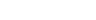As states with positive and negative values of ML are degenerate, we define
Λ = |ML|,

and a capital Greek letter is used to refer to each value: Λ = 0, 1, 2, 3... are coded as Σ, Π, Δ, Φ... respectively.
The molecular term symbol is then defined as
2S+1Λ

and the number of electron degenerate states (under the absence of spin-orbit coupling) corresponding to this term symbol is given by:
• (2S+1)×2 if Λ is not 0
• (2S+1) if Λ is 0.

## Ω and spin–orbit coupling

Spin–orbit coupling lifts the degeneracy of the electronic states. This is because the z-component of spin interacts with the z-component of the orbital angular momentum, generating a total electronic angular momentum along the molecule axis Jz. This is characterized by the MJ quantum number, where
MJ = MS + ML.

Again, positive and negative values of MJ are degenerate, so the pairs (ML, MS) and (−ML, −MS) are degenerate: {(1, 1/2), (−1, −1/2)}, and {(1, −1/2), (−1, 1/2)} represent two different degenerate states. These pairs are grouped together with the quantum number Ω, which is defined as the sum of the pair of values (ML, MS) for which ML is positive. Sometimes the equation
Ω = Λ + MS

is used (often Σ is used instead of MS). Note that although this gives correct values for Ω it could be misleading, as obtained values do not correspond to states indicated by a given pair of values (ML,MS). For example, a state with (−1, −1/2) would give an Ω value of Ω = |−1| + (−1/2) = −1/2, which is wrong. Choosing the pair of values with ML positive will give a Ω = 3/2 for that state.

With this, a level is given by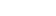Note that Ω can have negative values and subscripts r and i represent regular (normal) and inverted multiplets, respectively. For a 4Π term there are four degenerate (ML, MS) pairs: {(1, 3/2), (−1, −3/2)}, {(1, 1/2), (−1, −1/2)}, {(1, −1/2), (−1, 1/2)}, {(1, −3/2), (−1, 3/2)}. These correspond to Ω values of 5/2, 3/2, 1/2 and −1/2, respectively.
Approximating the spin–orbit Hamiltonian to first order perturbation theory
Perturbation theory (quantum mechanics)
In quantum mechanics, perturbation theory is a set of approximation schemes directly related to mathematical perturbation for describing a complicated quantum system in terms of a simpler one. The idea is to start with a simple system for which a mathematical solution is known, and add an...

, the energy level is given by
E = A ML MS

where A is the spin–orbit constant. For 4Π the Ω values 5/2, 3/2, 1/2 and −1/2 correspond to energies of 3A/2, A/2, −A/2 and −3A/2. Despite of having the same magnitude, levels of Ω = ±1/2 have different energies associated, so they are not degenerate. With that convention, states with different energies are given different Ω values. For states with positive values of A (which are said to be regular), increasing values of Ω correspond to increasing values of energies; on the other hand, with A negative (said to be inverted) the energy order is reversed. Including higher-order effects can lead to a spin-orbital levels or energy that do not even follow the increasing value of Ω.

When Λ = 0 there is no spin–orbit splitting to first order in perturbation theory, as the associated energy is zero. So for a given S, all of its MS values are degenerate. This degeneracy is lifted when spin–orbit interaction is treated to higher order in perturbation theory, but still states with same |MS| are degenerate in a non-rotating molecule. We can speak of a 5Σ2 substate, a 5Σ1 substate or a 5Σ0
substate. Except for the case Ω = 0, these substates have a degeneracy of 2.

## Reflection through a plane containing the internuclear axis

There are an infinite number of planes containing the internuclear axis and hence there are an infinite number of possible reflections. For any of these planes, molecular terms with Λ > 0 always have a state which is symmetric with respect to this reflection and one state that is antisymmetric. Rather than labelling those situations as, e.g., 2Π±, the ± is omitted.

For the Σ states, however, this two-fold degeneracy disappears, and all Σ states are either symmetric under any plane containing the internuclear axis, or antisymmetric. These two situations are labeled as Σ+ or Σ.

## Reflection through an inversion center: u and g symmetry

Taking the molecule center of mass as origin of coordinates, consider the change of all electrons' position from (xi, yi, zi) to (−xi, −yi, −zi). If the resulting wave function is unchanged, it is said to be gerade (German for even); if the wave function changes sign then it is said to be ungerade (odd). For a molecule with a center of inversion, all orbitals will be symmetric or antisymmetric. The resulting wavefunction for the whole multielectron system will be gerade if an even number of electrons is in ungerade orbitals, and ungerade if there is an odd number of electrons in ungerade orbitals, independently of the number of electrons in gerade orbitals.

## Alternative empirical notation

Electronic states are also often identified by an empirical single-letter label. The ground state is labelled X, excited states of the same multiplicity (i.e., having the same spin quantum number) are labelled in ascending order of energy with capital letters A,B,C...; excited states having different multiplicity than the ground state are labelled with lower-case letters a,b,c...
In polyatomic molecules (but not in diatomic) it is customary to add a tilde (e.g. X~, a~) to these empirical labels to prevent possible confusion with symmetry labels based on group representations.